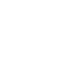in-the-given-figure-ab-and-ac-are-tangents-to-a-circle-with-centre-o-2Top matches
Q1.

In the given figure, AB and AC are tangents to a circle with centre O and radius 8 cm. If OA = 17 cm, then the length of AC (in cm) is

(a) 9    (b)  15   (c)  353  (d) 25Q2.
In given figure C is centre of circle. AO and BO are tangents to circle. CMAB.
If AC=AB=6cm, then find BC.Q3.
In the given figure,
AB and AC are tangents to the circle with centre O.
Given that BAC=70 and P is a point on the minor arc BC, find BPC.Q4.
In the given figure, common tangents AB and CD to the two circle with centres O1 and O2 intersect at E. Prove that AB = CD   [CBSE 2014]Q5.
In Figure 2, AB and AC are tangents to the circle with centre O such that BAC=40. Then BOC is equal to